Looking for strange particles in ALICE

1. Overview

The exercise proposed here consists of a search for strange particles, produced from collisions at LHC and recorded by the ALICE experiment. It is based on the recognition of their V0-decays, such as Kos → π+π-, Λ→ p + π- and cascades, such as Ξ- → Λ + π- ( Λ → p + π-). The identification of the strange particles is based on the topology of their decay combined with the identification of the decay products; the information from the tracks is used to calculate the invariant mass of the decaying particle, as an additional confirmation of the particle species.

In what follows the ALICE experiment and its physics goals are first presented briefly, then the physics motivation for this analysis. The method used for the identification of strange particles as well as the tools are described in detail; then all the steps of the exercise are explained followed by the presentation of the results; then all the steps of the exercise are explained followed by the presentation of the results as well as the method of collecting and merging all results. In the end the large scale analysis is presented.

2. Introduction.

ALICE (A Large Ion Collider Experiment), one of the four large experiments at the CERN Large Hadron Collider, has been designed to study heavy ion collisions. It also studies proton proton collisions, which primarily provide reference data for the heavy ion collisions. In addition, the proton collision data allow for a number of genuine proton proton physics studies. The ALICE detector has been designed to cope with the highest particle multiplicities anticipated for collisions of lead nuclei at the extreme energies of the LHC.

3. The ALICE Physics

Quarks are bound together into protons and neutrons by a force known as the strong interaction, mediated by the exchange of force carrier particles called gluons. The strong interaction is also responsible for binding together the protons and neutrons inside atomic nuclei.

Even though we know that quarks are elementary particles that build up all known hadrons, no quark has ever been observed in isolation: the quarks, as well as the gluons, seem to be bound permanently together and confined inside composite particles, such as protons and neutrons. This is known as confinement. The exact mechanism that causes it remains unknown.

Although much of the physics of strong interaction is, today, well understood, two very basic issues remain unresolved: the origin of confinement and the mechanism of the generation of mass. Both are thought to arise from the way the properties of the vacuum are modified by strong interaction.

The current theory of the strong interaction (called Quantum Chromo-Dynamics) predicts that at very high temperatures and very high densities, quarks and gluons should no longer be confined inside composite particles. Instead they should exist freely in a new state of matter known as quark-gluon plasma.

Such a transition should occur when the temperature exceeds a critical value estimated to be about 100 000 times hotter than the core of the Sun! Such temperatures have not existed in Nature since the birth of the Universe. We believe that for a few millionths of a second after the Big Bang the temperature was indeed above the critical value, and the entire Universe was in a quark-gluon plasma state.

When two heavy nuclei approach each other at a speed close to that of light and collide these extreme conditions of temperature are recreated and release the quarks and the gluons. Quarks and gluons collide with each other creating a thermally equilibrated environment: the quark–gluon plasma. The plasma expands and cools down to the temperature (10¹² degrees) at which quarks and gluons regroup to form ordinary matter, barely 10-23 seconds after the start of the collision. ALICE will study the formation and the properties of this new state of matter.

4. Strangeness enhancement as a signature for quark gluon plasma

The diagnosis and the study of the properties of quark-gluon plasma (QGP) can be undertaken using quarks not present in matter seen around us. One of the experimental signatures relies on the idea of strangeness enhancement. This was one of the first observables of quark-gluon plasma, proposed in 1980. Unlike the up and down quarks, strange quarks are not brought into the reaction by the colliding nuclei. Therefore, any strange quarks or antiquarks observed in experiments have been "freshly" made from the kinetic energy of colliding nuclei. Conveniently, the mass of strange quarks and antiquarks is equivalent to the temperature or energy at which protons, neutrons and other hadrons dissolve into quarks. This means that the abundance of strange quarks is sensitive to the conditions, structure and dynamics of the deconfined matter phase, and if their number is large it can be assumed that deconfinement conditions were reached.

In practice, the strangeness enhancement can be observed by counting the number of strange particles, that is particles containing at least one strange quark, and calculating the ratio of strange to non-strange particles. If this ratio is higher than that given by the theoretical models that do not foresee the creation of QGP, then enhancement has been observed.

For lead ion collisions, the number of strange particles is normalised to the number of nucleons participating in the interaction and compared with the same ratio for proton collisions.

5. Strange Particles

Strange particles are hadrons containing at least one strange quark. This is characterized by the quantum number of “strangeness”’. The lightest neutral strange meson is the Kos (ds) and the lightest neutral strange baryon is the Λ (uds), characterized as hyperon.

Here we will be studying their decays, for example Kos → π+π-, Λ→ p + π-. In these decays the quantum number of strangeness is not conserved, since the decay products are only composed of up and down quarks. Therefore these are not strong decays (which in addition would be very fast, with a τ = 10-23 s) but weak decays, in which the strangeness can be conserved (ΔS=0) or change by 1 (ΔS=1). For these decays the mean life τ is between 10-8 s and 10-10 s. For particles travelling close to the speed of light, this means that the particle decays at a distance (on average) of some cm from the point of production (e.g. from the point of the interaction).

6. How we look for strange particles

The aim of the exercise is to search for strange particles produced from collisions at LHC and recorded by the ALICE experiment.

As mentioned in the previous section, strange particles do not live long; they decay soon after their production. However, they live long enough to travel some cm distance from the interaction point (IP), where they were produced (primary vertex). Their search is thus based on the identification of their decay products, which must originate from a common secondary vertex.

Neutral strange particles, such as Kos and Λ, decay giving a characteristic decay pattern, called V0. The mother particle disappears some cm from the interaction point and two oppositely charged particles appear in its place, which are bent in opposite directions inside the magnetic field of the ALICE solenoid.

In the following red tracks indicate positively charged particles; green tracks indicate negatively charged particles.

The decays we will be looking for are: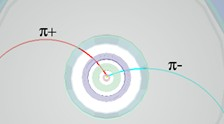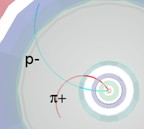Kos → π+π-Λ→ p + π-anti Λ→ p- + π+

We see that for a pion-pion final state the decay pattern is quasi-symmetric whereas in the pion-proton final state the radius of curvature of the proton is bigger than that of the pion: due to its higher mass the proton carries most of the initial momentum.

We will also be looking for cascade decays of charged strange particles, such as the Ξ-; this decays into π- and Λ; the Λ then decays into π- and proton; the initial pion is characterized as a bachelor (single charged track) and is shown in purple.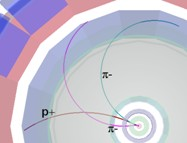Ξ-→π-Λ→ π- p + π-

The search for V0s is based on the decay topology and the identification of the decay products; an additional confirmation of the particle identity is the calculation of its mass; this is done based on the information (mass and momentum) of the decay products as described in the following section.

7. The (invariant) mass calculation

We consider the decay of the neutral kaon to two charged pions, Kos → π+π- .

Let E, p and m be the total energy, momentum (vector!) and mass of the mother particle (K0)
Let E1 , p1 and m1 be the total energy, momentum and mass of the daughter particle number 1(π+); and E2 , p2 and m2 the total energy, momentum and mass of the daughter particle number 2 (π-).

Conservation of energy E = E1 + E2 (1)
Conservation of momentum p = p1 + p2 (2)
From relativity (with the assumption c = 1) E2 = p2 + m2 (3)

Where p = |p| is the length or magnitude of the momentum vector p.

This applies, of course, also for the daughter particles

E12 = p12 + m12 (4)
E22 = p22 + m22 (5)

where p1 = |p2| and p2 = |p2| are the lengths of p1 and p2.

From the above equations, we find that:

m2 = E2 - p2 = (E1+E2)2 - (p1 + p2) 2
= E12 + E22 + 2E1E2 - p1.p2 - p2.p2 - 2 p1.p2 (6)

where we have introduced the scalar product p1.p2 of the two vectors p1 and p2, which is equal to the sum of the products of the x, y and z components of the two vectors:

p1.p2 = p1x p2x + p1y p2x + p1z p2z (7)
p1.p1 = p1x2 + p1y2 + p1z2 = p12 (8)
p1.p1 = p1x2 + p1y2 + p1z2 = p12 (9)

Equation (6) therefore becomes:

m2 = E12 + E22 + 2E1E2 - p12 - p22 - 2p1.p2
= m12 + m12 + 2E1E2 - 2p1.p2

We can therefore calculate the mass of the initial particle from the mass and the momentum components of the daughter particles.

The masses of the daughter particles m1 and m2 are known: a number of different detectors in ALICE identify particles.

We can therefore calculate the mass of the initial particle from the mass and the momentum components of the daughter particles.

The masses of the daughter particles m1 and m2 are known: a number of different detectors in ALICE identify particles.

The momenta of the daughter particles p1, p2 can be found by measuring the radius of curvature of their trajectory due to the known magnetic field. In the exercise we use the three components of the momentum vector of each track associated with the V0 decay, as in the above equations.

The invariant mass calculation gives typically distributions as shown below.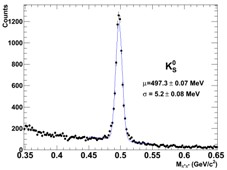The distribution on the left is the mass calculated for pion-proton pairs; the peak corresponds to Λ and the continuum is “background” from random combinations of pions and protons which appear as coming from the same secondary vertex or that have been misidentified; the distribution on the right is the mass calculated for negative and positive pion pairs; the peak corresponds to Kos.

8. The tools and how to use them

The exercise is done in the ROOT framework, using a simplified version of the ALICE event display: in a terminal window which is already open on your computer (so that you are in the appropriate directory) you type root masterclass.C. You will be presented with a small window, as shown in the picture.This offers the choice between demonstration mode, student mode for the event analysis and teacher mode for the collection and merging of the results.

The demo gives examples of Kos, Λ, anti-Λ and Ξ- decays.

The choice of “student mode” for the event analysis and visual search for V0s opens a window as shown in the next figure.

The column on the left offers a number of options: Instructions, Event Navigation, V0 and cascade finder, calculator, selection of what is displayed (tracks, detector geometry...). In addition, under “Encyclopedia”, there is a brief description of the ALICE detector and its main components, examples of the V0 decay patterns and examples of lead collision events.

The event display shows three views of the ALICE detector (3-dimensional view, rφ projection and rz projection). You can select the information displayed for each event. If you click on the relevant box, you see all the clusters and tracks of the event; if you click the V0 (and cascade) finder boxes, the V0s (and cascades) are highlighted, if they exist. Once a V0 is found, the rest of tracks and clusters of the event can be removed from the display so that only the tracks associated with the V0 are shown. The colour convention is that positive tracks from V0s are red, negative tracks are green (and “bachelors”, in the case of cascades, purple).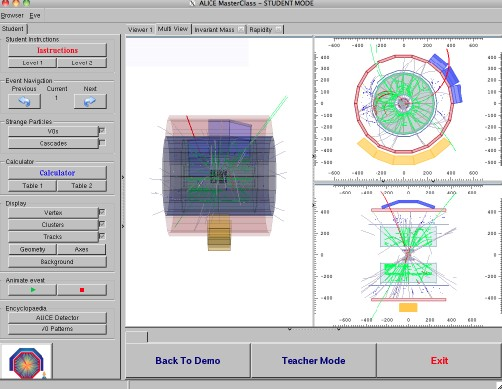By clicking on each track the values of the momentum components and the particle mass, (the one with the maximum probability, from the particle identification algorithms) appear on a little box (next figure, right). This information can be copied to the calculator, which then calculates the invariant mass of the mother particle, using the formula explained in the previous section.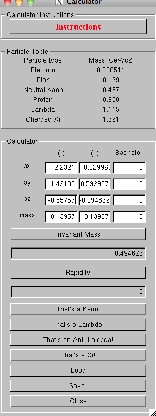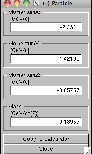The program includes four invariant mass histograms (for Kos, Λ, anti-Λ and Ξ-). After inspecting each V0 decay you can identify the mother particle from the decay products and the invariant mass value (a reference table with the masses of some particles is given as part of the calculator, see Figure). You then press the relevant button (That’s a kaon; that’s a Lambda etc.). In this way you add an entry to the corresponding histogram. The invariant mass histograms can be displayed by clicking on the invariant mass button, above the event display. To update their contents you must click inside each histogram.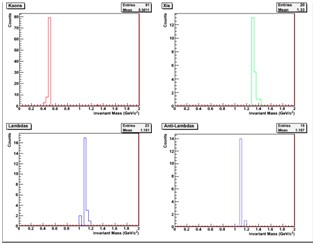9. The exercise - Analyse events and find the strange hadrons

The analysis part consists of the identification and counting of strange particles in a given event sample, typically containing 30 events. When starting the exercise, you should go to student mode and select the event sample that you will analyse. Currently there are 6 different event samples with data from proton proton collisions at 7 TeV centre-of-mass energy.

When looking at each event display, you initially have to click on the vertex, clusters and tracks; you can observe the complexity of the events and the high number of tracks produced by the collisions inside the detectors. Most of these tracks are pions.

By clicking on ‘V0’ and ‘Cascades’ the tracks from V0 decays - if any - and cascade decays –if any – appear highlighted. By clicking on each track you get the track information – the charge, the three components of the momentum vector and the mass of the most probable particle associated with the track. This has been found from the information provided by the different detectors used for Particle Identification (link). From the decay products you can already guess what the mother particle is; to confirm it, you calculate the invariant mass as described in section 7 and compare its value with the values on the table of your calculator.

If the mass is 497 MeV±13 MeV (in the interval [484, 510] MeV) it is a Kos;
If the mass is 1115 MeV ±5 MeV (in the interval [1110, 1120] MeV) and the daughter particles are a proton and a negative pion then it is a Λ.
If the mass is 1115 Me ± 5 MeV (in the interval [1110, 1120] MeV) and the daughter particles are an antiproton and a positive pion then it is an anti-Λ.
For a cascade decay, if the mass calculated from the 3 tracks is 1321± 10 MeV (in the interval [1311, 1331] MeV) then it is a Ξ-.

Depending on the outcome, you click on the button “It is a Kaon, Lambda etc”. In this way this entry is added in the corresponding invariant mass histogram.

It can happen that the calculated mass does not correspond to any of the above values; this is “background”: the tracks appear as coming from a secondary vertex, but in this case the vertex has been misidentified. For the purpose of this exercise we will ignore these V0’s.

10. Presentation of the results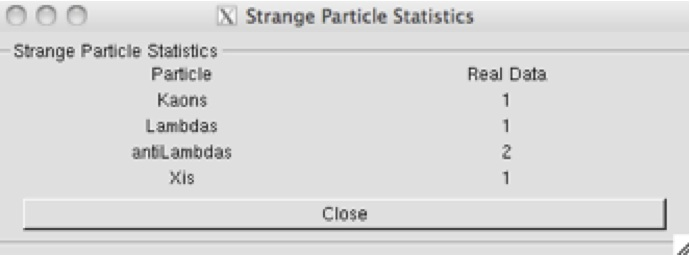This table summarises the results. The column "real data" contains the numbers of Kos, Λ, anti-Λ and Ξ- that you found (provided you remembered to press the “This is a Kaon, Lambda etc“ button).

You can also look at the invariant mass histograms and check the number of entries for each particle type. When you have analysed all events in your data sample, save the results on a file following the instructions inside the analysis program.

11. Collection of all results

Selecting the option “Teacher” in the initial MasterClass menu, you can collect all the results. Under “Teacher Controls” you select the Get Files option and get, one at a time, the files with the analysis results from each data sample. Obviously you need to transfer the files with the results to the “Teacher’s” computer first! Then, under “Results”, you can look at the table which now contains the full statistics.

12. Large statistics analysis

The event display is a powerful tool which helps check the quality of the data and their reconstruction and gives a “feeling” of what events look like. However in real life data analysis is not done visually –that would be far too tedious and time-consuming. To analyse the millions of events that we collect daily at the LHC we run programs, and this is what you will do here, in order to look for V0s in a bigger event sample.

On your terminal window change directory (by giving the command: cd MasterClass_extended) and type root MasterClassExtended.C. In the “put your name here” space, give a combination of characters which will form the name of the results file. Choose a data sample to analyse (currently there is a sample with pp data and a sample with PbPb data); then choose “student” to proceed to the analysis.

Under “Analysis tools” you can analyse 100 events or all events in your data sample and calculate the invariant mass of pairs of particles, such as π+π-. You can see that the invariant mass is a continuous distribution – this is because the pairs of pions combined are random, not coming from a common secondary vertex and can give any value of mass. This is the background.

When you proceed to the V0 selection, only pairs of tracks coming from a common secondary vertex are considered; their invariant mass is calculated based on the track information and the mass of the identified decay products. You can select Kos or Λ (including anti-Λ). Each time the analysis of all events in the sample is finished (watch the terminal behind the menu) the invariant mass distribution is displayed on the screen.

In order to find the number of particles of a certain type, for example Kos, you need to find the number of events in the peak after background subtraction. In order to fit a curve (second degree polynomial) to the background, you first choose the fit range using the slider. To fit a Gaussian distribution to the signal you also have to select the range of the peak. When you click on “Fit signal and background”, the fitted functions are superimposed on the histogram and you can check visually whether the fit is reasonable. For the background subtraction the coefficients of the second degree polynomial are used; on the histogram you get the total number of events in the peak, the number of background events and those which are signal, as well as the mean value of the Gaussian (the particle mass) and its width sigma (both in MeV/c2).

13. Find Kos Λ, anti-Λ in PbPb collisions in different centrality regions

When studying heavy ion collisions we often classify the events in different classes, according to the centrality of the collision. The lead nucleus is much bigger than the proton and this leads to differences in how the particles collide. In the case of proton-proton there is only one kind of collision while lead collisions are differentiated by the size of the region of each nucleus that overlap. To see the difference between proton-proton and lead-lead collisions we first define different event classes for heavy ion collisions. One of the criteria used is the collision centrality, which is related to the impact parameter (the distance between the colliding nuclei perpendicular to the beam axis). However this parameter is not directly measurable. One of the ways in which ALICE determines the centrality of the events is from the signal amplitude in the VZERO counters (2 arrays of plastic scintillators placed at +330 cm and -90 cm from the interaction point). This is shown in the right-hand figure. Quantitative estimate of the collision centrality is given by the number of participating nucleons Npart (shown in red in the left-hand figure).In this part of the exercise you will analyse event samples belonging to different centrality classes looking for V0s and calculate the number of Kos, Λ, anti-Λ for each centrality class. In the directory MasterClass_cent you have to type root MasterClassExtended.C . You select “student” and in the new panel which appears you select the V0 type and the centrality class by clicking on the relevant button. Once you have the invariant mass spectrum, you find the number of particles of a certain species by following the procedure for fitting background and signal described in the previous section.

14. Calculation of particle yields

Once you have found the number of Kos, Λ, anti-Λ you can calculate the yield (number of particles produced per interaction) for each type of V0. To do this you need additional information which will be given to you after you have finished the analysis (for example : tracking efficiency for each type of particle; number of events from which the sample you analyse has been extracted).